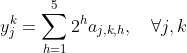# Print infeasibility report using cvx mosek

My code generates an infeasible solution when I used mosek as solver in cvx matlab. I want to print the infeasibility report so that I can find where the error is happening.

Make sure that quiet mode is not specified . Then the solver log steam will be shown.

To specify that a short infeasibility report be printed to the log stream, use the command
cvx_solver_settings('MSK_IPAR_INFEAS_REPORT_AUTO','MSK_ON")
or
cvx_solver_settings('MSK_IPAR_INFEAS_REPORT_AUTO','MSK_ON','MSK_IPAR_INFEAS_REPORT_LEVEL',n)
to specify output level n.

However, the Mosek infeasibility report provided in the log stream will be for the problem provided to Mosek, after whatever transformations CVX has applied to your problem. It may not be obvious how that relates to the problem as you entered it into CVX. That is a down side to using a tool such as CVX.

CVX usually sends the dual problem to Mosek it will say at the beginning of the output if this is the case.if it does, Mosek’s primal infeasibility report pertains to unboundedness of the primal. if Mosek is solving the dual, you need to look at Mosek’s dual infeasibility report to get information on the primal, as you entered the problem.

I will defer any more advanced discussion of this to the Mosek developers who read this forum.

Thank you @Mark_L_Stone . It seems I have error in one of the constraints. Can you help me with representing the below constraint: y_j^k = \sum_{h=1}^{5} 2^h a_{j,k,h} ∀j,k
% Integer to binary conversion
for j = 1:J
for k = 1:K
y(j,k) == sum(2^h * a(j,k,h));
end
end

I either get elementwise matrix power error or elementwise multiplication error. I tried fixing both ways but didn’t work.

I don’t know what are CVX variables or expression, as opposed to input data. So I don’t even know whether this is a convex constraint. And perhaps you want y to be an expression holder, rather than specifying constraints. You need t provide more information.

Is CVX accepting your problem, and you are dissatisifed with the result? or does the above code produce an error message as son as it is entered?

Both y and a are cvx variables of the following dimensions:
variable y(5,4) integer
variable a(5,4,5) binary

When I run my entire code this is the result:
Status: Infeasible
Optimal value (cvx_optval): -Inf
However when I was verifying if my individual constraints were as per requirement, I found out that the summation over h wasn’t being done in the below mentioned constraint, instead it was checking for every j,k,h.Maybe if this is fixed the infeasibility would go, considering there are no other errors for now. If you can help me with this I can verify my code further. Thanks again @Mark_L_Stone

Perhaps you want code such as this. I’m not sure this is quite right, but I’ll let you check it, and fix it up as necessary.

h = (1:5)';
y == sum(repmat(2.^h,1,4,5).*a,3)

I checked the code.
The result generated is as follows for j=k=1 and h=1:5
“y(1, 1) - 2a(1, 1, 1) - 2a(1, 1, 2) - 2a(1, 1, 3) - 2a(1, 1, 4) - 2a(1, 1, 5) == 0"
However I desire the result to be:
"y(1, 1) - 2
a(1, 1, 1) - 4a(1, 1, 2) - 8a(1, 1, 3) - 16a(1, 1, 4) - 32a(1, 1, 5) == 0”

It tried to vertically stack the row vector five times. I am quite new to use of repmat, I did check the matlab help center but wasn’t successful in generating the desired output. Could you help me with this or maybe suggest some links?

I did say you need to check it and fix it up.

I think changing the 3 to 1 might do the trick.

h = (1:5)’;
y == sum(repmat(2.^h,1,4,5).*a,1)

Changing to 1 , generates the following error:
Argument dimensions 5-by-4 and 1-by-4-by-5 must agree.

I will try fixing it. Thanks for you help @Mark_L_Stone

This should work:

h=(1:5)';
a=ones(5,4,5); % test data
y == reshape(sum(repmat(2.^h,1,4,5).*a,1),5,4)


disp(size(reshape(sum(repmat(2.^h,1,4,5).*a,1),5,4)))
5 4